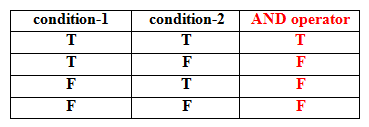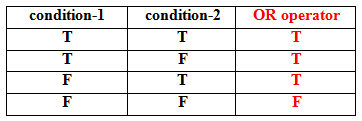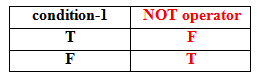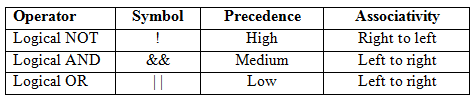## Logical operators

View

These operators are used to combine two conditions. Various logical operators in C are-

1. Logical AND operator – Symbol - &&
2. Logical OR operator – Symbol - | |
3. Logical NOT operator – Symbol - !
Out of these Logical AND and Logical OR are binary operators whereas Logical NOT is an unary operator.

Logical AND operator

Syntax-

(condition-1) AND operator (condition-2)

This operator returns TRUE when both the conditions are TRUE, otherwise it returns FALSE.

Truth TableExample-1

Let a =10, b = 20, c = 30

(a > b) && (a > c)                                          //          False
(b > a) && (b > c)                                          //          False
(c > a) && (c > b)                                          //          True

Example-2

Let a =10, b = 20, c = 30, d =40

(a > b) && (a > c) && (a > d)                                               //          False
(b > a) && (b > c) && (b > d)                                              //          False
(c > a) && (c > b) && (c > d)                                               //          False
(d > a) && (d > b) && (d > c)                                              //          True

Logical OR operator

Syntax-

(condition-1) OR operator (condition-2)

This operator returns TRUE when any of both the conditions are TRUE. If both the conditions are FALSE it returns FALSE.

Truth TableExample-1

Let a =10, b = 20, c = 30

(a > b) | | (a > c)                                              //          False
(b > a) | | (b > c)                                              //          True
(c > a) | | (c > b)                                              //          True

Example-2

Let a =10, b = 20, c = 30, d =40

(a > b) | | (a > c) | | (a > d)                                           //          False
(b > a) | | (b > c) | | (b > d)                                          //          True
(c > a) | | (c > b) | | (c > d)                                           //          True
(d > a) | | (d > b) | | (d > c)                                          //          True

Logical NOT operator

Syntax-

NOT operator (condition-1)

This operator returns TRUE when condition is FALSE and FALSE when condition is TRUE

Truth TableExample-1

Let a =10, b = 20

!(a > b)                                                //          True
!(b > a)                                                //          False

Mixing of Logical operatorsPrecedence order of Logical operators and associativity

Example-

Let condition a =True, b = False, c = True, d =False

a | | b && c                          means-            a | | (b && c)                        output-True
a && b | | c && d                 means-            (a && b) | | (c && d)             output-False
a && b && c | | d                 means-            ((a && b) && c) | | d             output-False
!a && b | | c                         means-            (( !a ) && b) | | c                   output-True

Example-3

Marks of 5 subjects are input through the keyboard. Write a Program in C to print the total marks, percentage and division.

/* Program to display total marks, percentage and division [division.c] */
#include < stdio.h >
#include < conio.h >
void main ( )
{
// Variable Declaration
int s1, s2, s3, s4, s5, total ;
float per ;
clrscr ( );

// Input
printf ( “ \n Enter the marks of Physics \n “ );
scanf ( “ %d “, &s1 );
printf ( “ \n Enter the marks of Chemistry \n “ );
scanf ( “ %d “, &s2 );
printf ( “ \n Enter the marks of Maths \n “ );
scanf ( “ %d “, &s3 );
printf ( “ \n Enter the marks of English \n “ );
scanf ( “ %d “, &s4 );
printf ( “ \n Enter the marks of Computer Science \n “ );
scanf ( “ %d “, &s5 );

// Processing and Output
total = s1 + s2 + s3 + s4 +s5 ;
per = total / 5.0 ;
printf ( “ \n Total Marks = %d“, total );
printf ( “ \n Percentage = %f “, per );
if ( per > = 75 )
printf ( “ \n First Division with Distinction \n “ );
if ( per > = 60 && per < 75)
printf ( “ \n First Division \n “ );
if ( per > = 45 && per < 60)
printf ( “ \n Second Division \n “ );
if ( per > = 33 && per < 45)
printf ( “ \n Third Division \n “ );
if ( per < 33 )
printf ( “ \n Fail \n “ );
getch ( );
}